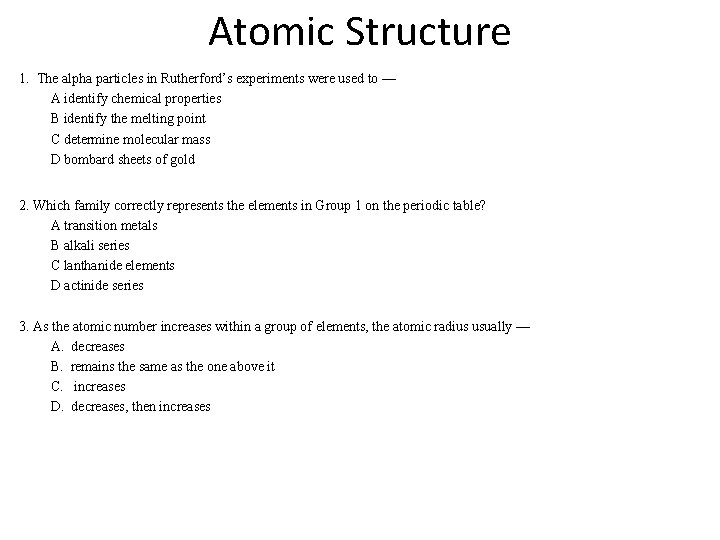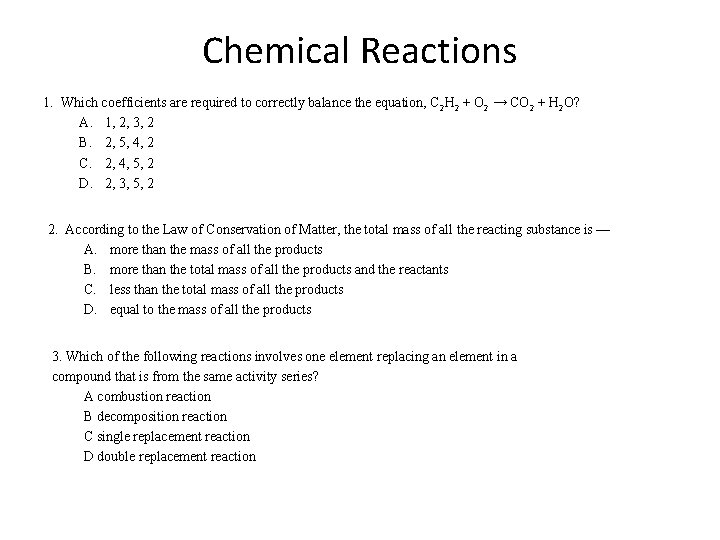# Density Solve the following problems 1 A block

• Slides: 8
Download presentationDensity Solve the following problems: 1. A block of maple wood with a volume of 405 cubic centimeters and a density of. 67 g/cm 3 is sawed in half. The density of the two smaller blocks is now — A one-fourth the original density B one-half the original density C two times the original density D the same as the original density 2. What is the mass of a 500. 00 m. L sample of seawater with a density of 1. 025 g/m. L? A. 487. 8 g B. 500. 0 g C. 512. 5 g D. 625. 0 g 3. What is the density at 20°C of 12. 0 milliliters of a liquid that has a mass of 4. 05 grams? A. B. C. D. 0. 338 g/m. L 2. 96 g/m. L 16. 1 g/m. L 48. 6 g/m. LLab Management 1. A student spills a chemical in the laboratory. What should he/she do first? A Clean it up. B Tell the teacher. C Let it evaporate. D Dilute the spill with water. 2. Eating in the laboratory — A. is not permitted B. is permitted if it is purchased in the cafeteria C. is permitted if the lab experiment does not involve chemicals D. is permitted as soon as the lab experiment is finished 3. What should be used to put out a fire in a person’s hair or clothing? A your hands B a fire blanket C a fire extinguisher D water from the sink faucetMatter 1. Which of the following is considered to be a chemical property of matter? A. viscosity B. reactivity C. texture D. density 2. Which of the following is a CORRECT statement regarding mixtures? A. Mixtures are composed of a single substance. B. Mixtures are composed of more than one substance. C. Mixtures have a fixed composition. D. All substances in a mixture are visible at all times. 3. An object is observed to have a silver color and a metallic appearance. The object is also found to have a mass of 11. 34 grams and melts at 327. 46°C. Of the observed properties listed below, which is considered to be an extensive property? A. silver B. metallic C. mass D. melting pointAtomic Structure 1. The alpha particles in Rutherford’s experiments were used to — A identify chemical properties B identify the melting point C determine molecular mass D bombard sheets of gold 2. Which family correctly represents the elements in Group 1 on the periodic table? A transition metals B alkali series C lanthanide elements D actinide series 3. As the atomic number increases within a group of elements, the atomic radius usually — A. decreases B. remains the same as the one above it C. increases D. decreases, then increasesChemical Bonding 1. What is the electron configuration for manganese, atomic number 25? A 1 s 2 2 p 6 3 s 2 3 p 6 4 s 2 3 d 5 B 1 s 2 2 p 6 3 s 2 3 p 6 4 s 2 3 d 10 C 1 s 2 2 p 6 3 s 2 3 p 6 4 s 2 D 1 s 2 2 p 6 3 s 2 3 p 6 2. A property of some metals is the ability to be drawn into a wire. This is called — A malleability B ductility C permeability D conductivity 3. Which of the 2 outermost orbitals MUST BE FILLED to satisfy the octet rule? A d and f orbitals B d and p orbitals C s and d orbitals D s and p orbitalsChemical Formulas 1. What is the formula for barium nitrate? A. Ba(NO 3)2 B. Ba. NO 2 C. Ba 2 NO 3 D. Ba(NO 4)2 2. What is the name of the compound Al. PO 4? A aluminum phosphide B aluminum phosphate C aluminum phosphite D aluminum phosphoroxide 3. What is the name of the compound N 2 O 3? A. sodium dioxide B. dinitrogen oxide C. nitrous oxide D. dinitrogen trioxideThe Mole 1. How many Mg 2+ ions are found in 1. 00 mol of Mg. O? A. 3. 01 x 1023 B. 12. 04 x 1023 C. 6. 02 x 1023 D. 6. 02 x 1025 2. How many grams of Na. Cl are present in 11. 00 moles? A. 0. 4500 g B. 156. 6 g C. 331. 0 g D. 642. 9 g 3. What is the molar mass of Mg. SO 4? A 116. 13 amu B 272. 83 amu C 111. 21 amu D 120. 37 amuChemical Reactions 1. Which coefficients are required to correctly balance the equation, C 2 H 2 + O 2 → CO 2 + H 2 O? A. 1, 2, 3, 2 B. 2, 5, 4, 2 C. 2, 4, 5, 2 D. 2, 3, 5, 2 2. According to the Law of Conservation of Matter, the total mass of all the reacting substance is — A. more than the mass of all the products B. more than the total mass of all the products and the reactants C. less than the total mass of all the products D. equal to the mass of all the products 3. Which of the following reactions involves one element replacing an element in a compound that is from the same activity series? A combustion reaction B decomposition reaction C single replacement reaction D double replacement reaction